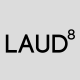Not knowing what to call this routine, I think that it is more of a combination of an advanced Offset command that automates the placement of the vertices at the (M2P) Mid-Between-2-Points.

If you need an offset that is between 2 polylines and the 2 polylines are not truly parallel to each other, the result of the polyline might not be the desired result.This process is automated by using this routine written by Alan Thompson and found at CADTutor: http://www.cadtutor.net/forum/showthread.php?73308-Multiple-Offsets&p=535978&viewfull=1#post535978

(Please refer questions or requests to the forum where the lisp routine was originally posted)Note: that the output offers an option for the created polyline (LWPolyline or Polyline)As an added bonus, the routine handles polylines that are at different elevations (z values) and it even handles 3DPolylines pretty well.Thanks Alan!!

Here’s how:

• LBL <enter> to start
• Select the 1st polyline
• Select the 2nd polyline
• Specify the output polyline (LWPoline or Polyline)
• RE <enter> or REGEN <enter> to get rid of the temporary dashed red lines

```
(defun c:LBL (/ foo AT:GetSel _pnts _pline _lwpline _dist e1 e2)
;; Draw (LW)Polyline between two selected curves (at midpoint of vertices).
;; Alan J. Thompson, 09.29.10

(defun foo (e)
(and (wcmatch (cdr (assoc 0 (entget (car e)))) "LINE,*POLYLINE,SPLINE")
(not (vlax-curve-isClosed (car e)))
)
)

(defun AT:GetSel (meth msg fnc / ent)
;; meth - selection method (entsel, nentsel, nentselp)
;; msg - message to display (nil for default)
;; fnc - optional function to apply to selected object
;; Ex: (AT:GetSel entsel "\nSelect arc: " (lambda (x) (eq (cdr (assoc 0 (entget (car x)))) "ARC")))
;; Alan J. Thompson, 05.25.10
(while
(progn (setvar 'ERRNO 0)
(setq ent (meth (cond (msg)
("\nSelect object: ")
)
)
)
(cond ((eq (getvar 'ERRNO) 7) (princ "\nMissed, try again."))
((eq (type (car ent)) 'ENAME)
(if (and fnc (not (fnc ent)))
(princ "\nInvalid object!")
)
)
)
)
)
ent
)

(defun _pnts (e / p l)
(if e
(cond ((wcmatch (cdr (assoc 0 (entget e))) "ARC,LINE,SPLINE")
(list (vlax-curve-getStartPoint e) (vlax-curve-getEndPoint e))
)
((wcmatch (cdr (assoc 0 (entget e))) "*POLYLINE")
(repeat (setq p (1+ (fix (vlax-curve-getEndParam e))))
(setq l (cons (vlax-curve-getPointAtParam e (setq p (1- p))) l))
)
)
)
)
)

(defun _pline (lst)
(if (and (> (length lst) 1)
(entmakex '((0 . "POLYLINE") (10 0. 0. 0.) (70 . 8)))
(foreach x lst (entmakex (list '(0 . "VERTEX") (cons 10 x) '(70 . 32))))
)
(cdr (assoc 330 (entget (entmakex '((0 . "SEQEND"))))))
)
)

(defun _lwpline (lst)
(if (> (length lst) 1)
(entmakex (append
(list '(0 . "LWPOLYLINE")
'(100 . "AcDbEntity")
'(100 . "AcDbPolyline")
(cons 90 (length lst))
(cons 70 (* (getvar 'plinegen) 128))
)
(mapcar (function (lambda (p) (list 10 (car p) (cadr p)))) lst)
)
)
)
)

(defun _dist (a b) (distance (list (car a) (cadr a)) (list (car b) (cadr b))))

(if
(and
(setq e1 (_pnts (car (AT:GetSel entsel "\nSelect first open curve: " foo))))
(setq e2 (_pnts (car (AT:GetSel entsel "\nSelect next open curve: " foo))))
(not (initget 0 "Lwpolyline Polyline"))
(setq *LBL:Opt* (cond ((getkword (strcat "\nSpecify line to draw: [Lwpolyline/Polyline] <"
(cond (*LBL:Opt*)
((setq *LBL:Opt* "Lwpolyline"))
)
">: "
)
)
)
(*LBL:Opt*)
)
)
)
((if (eq *LBL:Opt* "Lwpolyline")
_lwpline
_pline
)
(vl-remove nil
(mapcar (function (lambda (a b)
(if (and a b (not (grdraw (trans a 0 1) (trans b 0 1) 1 1)))
(mapcar (function (lambda (a b) (/ (+ a b) 2.))) a b)
)
)
)
e1
(if (< (_dist (car e1) (car e2))
(_dist (car e1) (last e2))
)
e2
(reverse e2)
)
)
)
)
)
(princ)
)
```This blog serves as a knowledge base for myself (and anyone else) so that I can reference tips & tricks that I have learned and also refer others to it as well. I hope that this blog helps you learn at least one tip to make your drafting/design experience better.
This entry was posted in AutoLISP, AutoLISP: Creating, AutoLISP: Modify, AutoLISP: Polylines. Bookmark the permalink.

### 4 Responses to AutoLISP: Advanced Polyline Offset

1.Doug says:

This is terrific. Thanks!

2.Szigeti Pál says:

Does it work on polylines with different number of points?

3.laud8 says:

You always make me learn a useful thing! Thanks Greg! :)

•AutoCAD Tips says:

Thanks for the compliment. I find your blog inspiring. Blending art and architecture and especially landscape architecture which I think is some of the hardest architecture to pull off and make it really stand out. You guys do a great job.
Thanks
~Greg